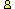CTK Exchange Front Page Movie shortcuts Personal info Awards Reciprocal links Terms of use Privacy Policy Cut The Knot! MSET99 Talk Games & Puzzles Arithmetic/Algebra Geometry Probability Eye Opener Analog Gadgets Inventor's Paradox Did you know?... Proofs Math as Language Things Impossible My Logo Math Poll Other Math sit's Guest book News sit's Recommend this siteCTK Exchange

 Subject: "Modular arithmetic" Previous Topic | Next Topic
 ConferencesThe CTK ExchangeThis and thatTopic #766Printer-friendly copyEmail this topic to a friend Reading Topic #766
Monty
Member since Jul-27-04
May-12-07, 10:25 PM (EST)"Modular arithmetic"

 The definition of mod is, I understand, if a = b mod n, then (a-b) is divisible by n, or a-b=kn, with k an integer I'm applying this to Fermat's little theorem: so if a^(p-1) = 1 mod p, then a^(p-1)-1 = kp multiplying both sides by a, we get a^p - a = kap = cpSo a^p - a = cp and a^(p-1)-1 = kp would seem to be equivalent.HOWEVER, they seem NOT equivalent if a=p a=p=2, 2^2-2=2=1*2, but 2^1-1=1 not a product of 2 a=p=3, 3^3-3=27-3=24=8*3, but 3^2-1=9-1=8 not a product of 3Why is this?It would seem that I'm somehow dividing by a-p, which is of course NG if a=p. But I don't see where.... ??? Monty

alexbCharter Member
2009 posts
May-13-07, 08:04 AM (EST)1. "RE: Modular arithmetic"
In response to message #0

 If a is divisible by p then a = 0 (mod p). So, in this case, multipliying by a is multiplying by 0.Montyguest
May-15-07, 12:29 PM (EST)

2. "RE: Modular arithmetic"
In response to message #1

 I see what you're saying, but still don't understand. From the definition of mod I got an equation which said a^(p-1)-1=kp, that is it equals a product of p. I then multiplied by a and got a^p-a=cp, so it's also a product of p. If a=0 (mod p), then a=fp -- a is a product of p. So my second equation is fp^p-fp=cp. Let's see, I can factor out p, so I get fp^(p-1)-f=c. Now the function y=x^(x-1)+k is a little irregular (when x is negative....), but otherwise it's an ok function.So I'm still missing the point.alexbCharter Member
2009 posts
May-15-07, 12:39 PM (EST)3. "RE: Modular arithmetic"
In response to message #2

 >I see what you're saying, but still don't understand. From >the definition of mod I got an equation which said >a^(p-1)-1=kp, This is only true if a is coprime with p.>I then >multiplied by a and got a^p-a=cp, This is true for any a, for if p|a the fact is trivial.>so it's also a product of p. If a=0 (mod p), then a=fp -- a is a product of p. So my >second equation is fp^p-fp=cp. This is trivially true, yes. >Let's see, I can factor out >p, so I get fp^(p-1)-f=c. Now the function y=x^(x-1)+k is a >little irregular (when x is negative....), but otherwise >it's an ok function. >>So I'm still missing the point. I am not sure what point it is, so I am going to check your original message.

alexbCharter Member
2009 posts
May-15-07, 12:42 PM (EST)4. "RE: Modular arithmetic"
In response to message #0

 >The definition of mod is, I understand, > if a = b mod n, then (a-b) is divisible by n, or a-b=kn, >with k an integer > I'm applying this to Fermat's little theorem: > so if a^(p-1) = 1 mod p, then a^(p-1)-1 = kp > multiplying both sides by a, we get > a^p - a = kap = cp >So a^p - a = cp and a^(p-1)-1 = kp would seem to be >equivalent. In a sense, yes, but you must be cautious as to the meaning of equivalence.a^(p-1)-1 = kp holds only for a coprime with p, whereasa^p - a = cp holds for any a,

 Conferences | Forums | Topics | Previous Topic | Next Topic
 Select another forum or conference Lobby The CTK Exchange (Conference)   |--Early math (Public)   |--Middle school (Public)   |--High school (Public)   |--College math (Public)   |--This and that (Public)   |--Guest book (Protected)   |--Thoughts and Suggestions (Public) Educational Press (Conference)   |--No Child Left Behind (Public)   |--Math Wars (Public)   |--Mathematics and general education (Public)You may be curious to have a look at the old CTK Exchange archive.